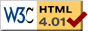### Journée-séminaire de combinatoire

#### (équipe CALIN du LIPN, université Paris-Nord, Villetaneuse)

Le 15 février 2011 à 14h00 en B311, Alexandre Benoit nous parlera de : Expansions de Fourier et de Chebyshev de fonctions D-finies

Résumé : Chebyshev polynomials, Hermite polynomials, Bessel functions and other families of special functions each form a basis of some Hilbert space. A Generalized Fourier Series is a series expansion in one of these bases, for instance a Chebyshev series. When such a series solves a linear differential equation, its coefficients satisfy a linear recurrence equation. We interpret this equation as the numerator of a fraction of linear recurrence operators. This interpretation lets us give a general algorithm for computing this recurrence, and a simple view of existing algorithms for several specific function families. Joint work with Bruno Salvy.

 Dernière modification : Monday 24 January 2022Contact pour cette page : Cyril.Banderier at lipn.univ-paris13.fr